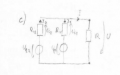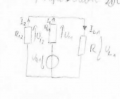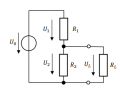How can I know if I am dealing with a loaded voltage divider.

arhzz1

Joined Oct 21, 2020
42
Hello!

Consider this circuit;Now the values of the voltage sources and resistors are not really important.What I want to find is the Voltage at the resitor R;

Now I tried this; Since I have two voltage sources I used the superpostion theorem.I simply removed one of the voltage sources and left the other one active. I first deactived the left one ( Uq2) and this is how my circuit looks now.Now this is (according to the solution sheet) a loaded voltage divider.And I am not really sure I understand how?What is specifically bothering me is that I am used to seeing the voltage divider in this form;Now the way I interprate my second picture is that the voltage source is underneath the R2 in this picture,and I cant move it to the left since there is a node,and I cant push Uo over the node.

Could anyone give me insight on how I should see the voltage divider when the position of the voltage source is a bit "off" the usual? What tip could you give to determine it without having to look alike a "normal form"

Thanks

ericgibbs

Joined Jan 29, 2010
14,715

ericgibbs

Joined Jan 29, 2010
14,715
hi arh.
This is your circuit redrawn [which can often help analysis]

Note: your circuit does not show any common reference node.
E

arhzz1

Joined Oct 21, 2020
42
hi arh,
Looking at your original circuit, which method are you using to solve this Network problem.?
E
View attachment 253499
Hi eric,

Your redrawing of my circuit helps a ton.Thank you very much.

Im using the superposition theorem to solve this circuit

ericgibbs

Joined Jan 29, 2010
14,715
Hi arh.
As I said, redrawing a network can make it easier to visualize the problem/solution.E